Constructing Perpendicular bisector

Practical Geometry
Serial order wise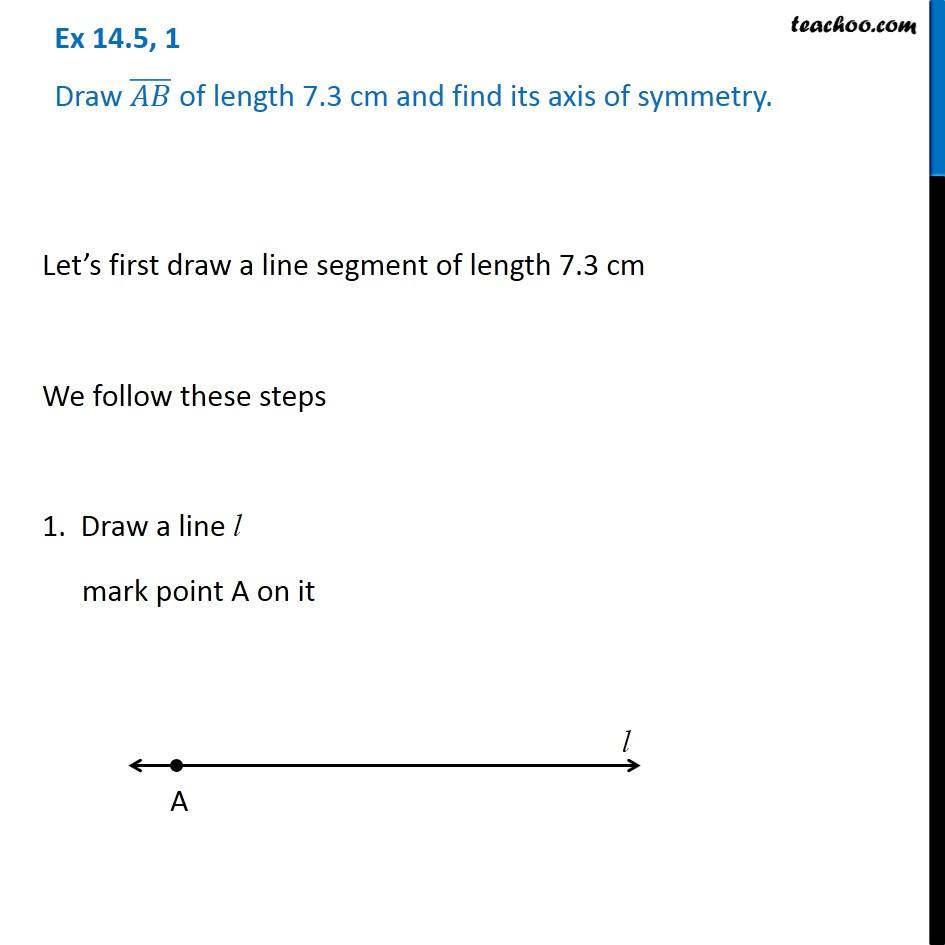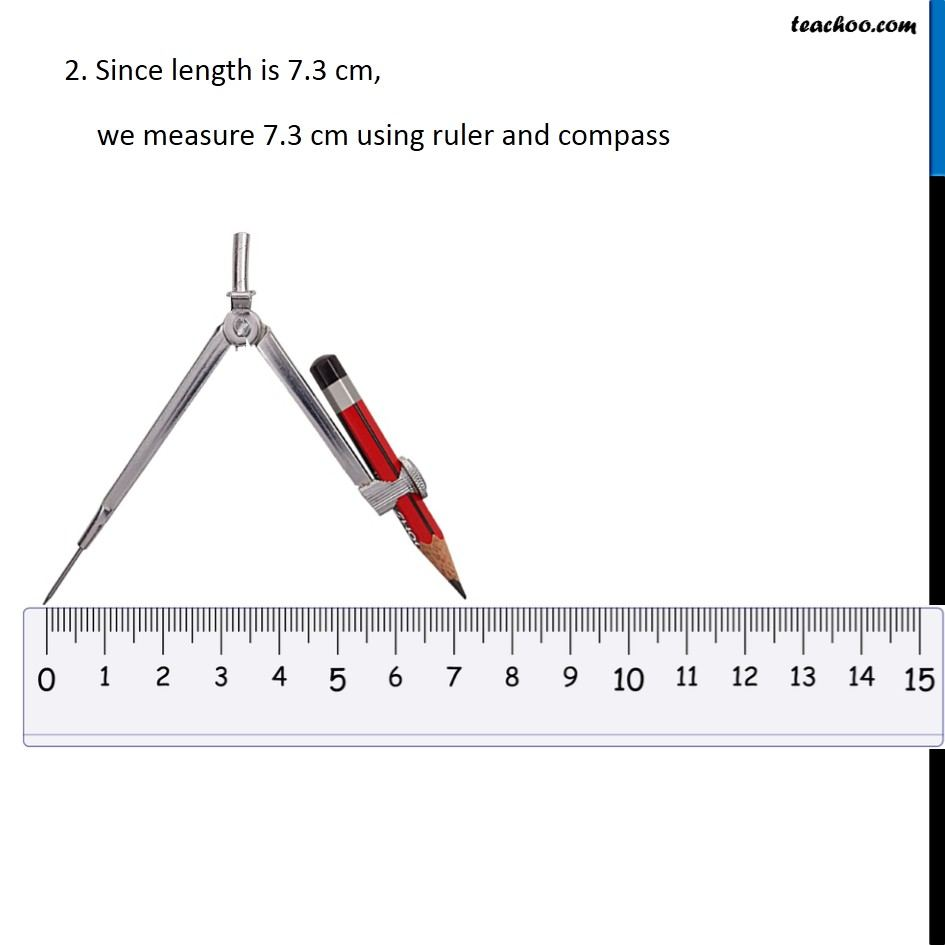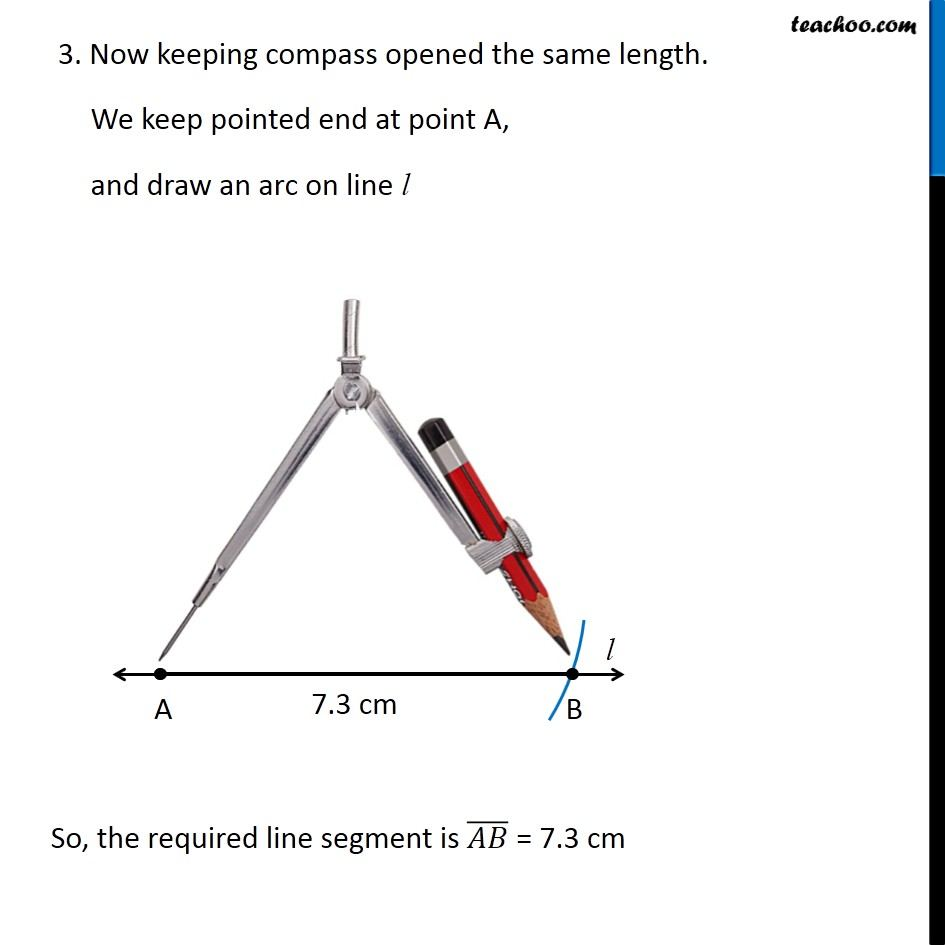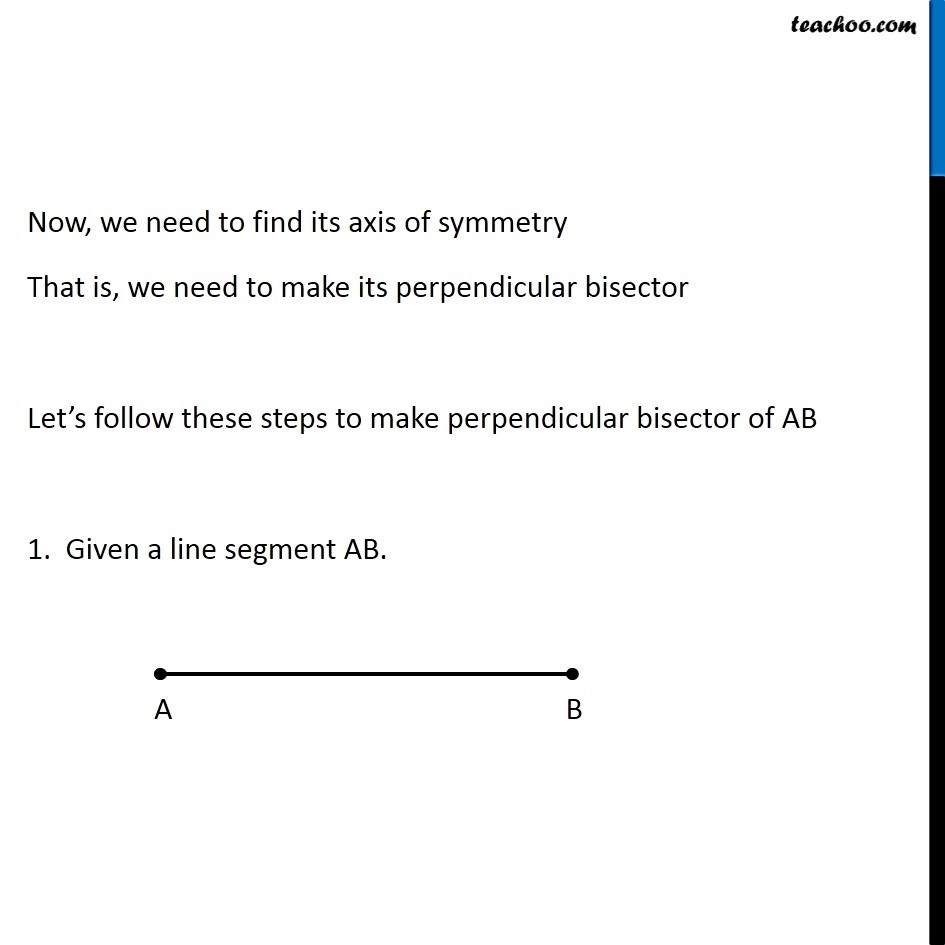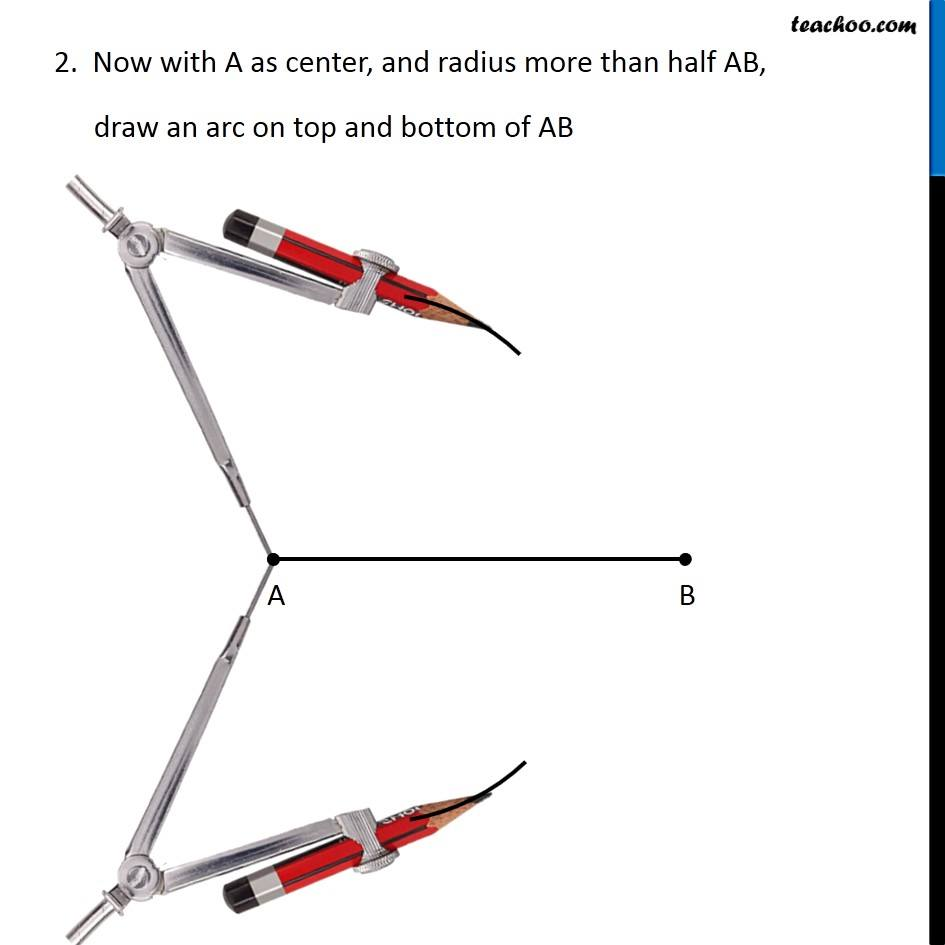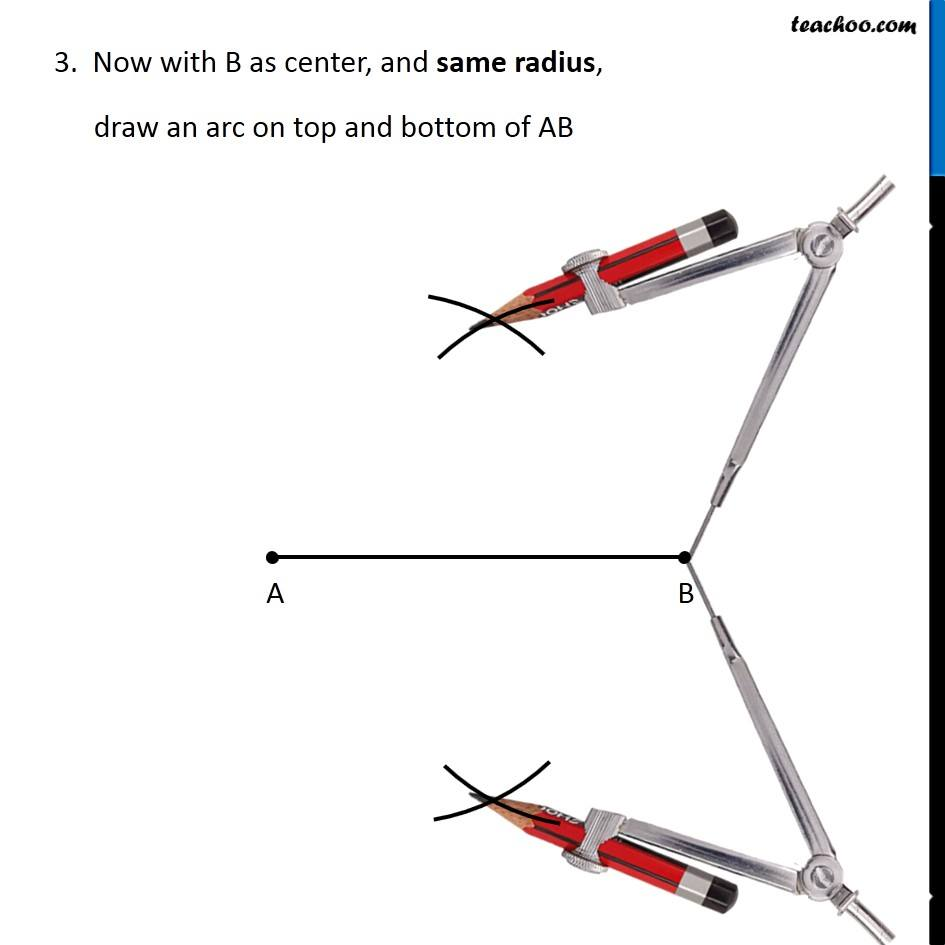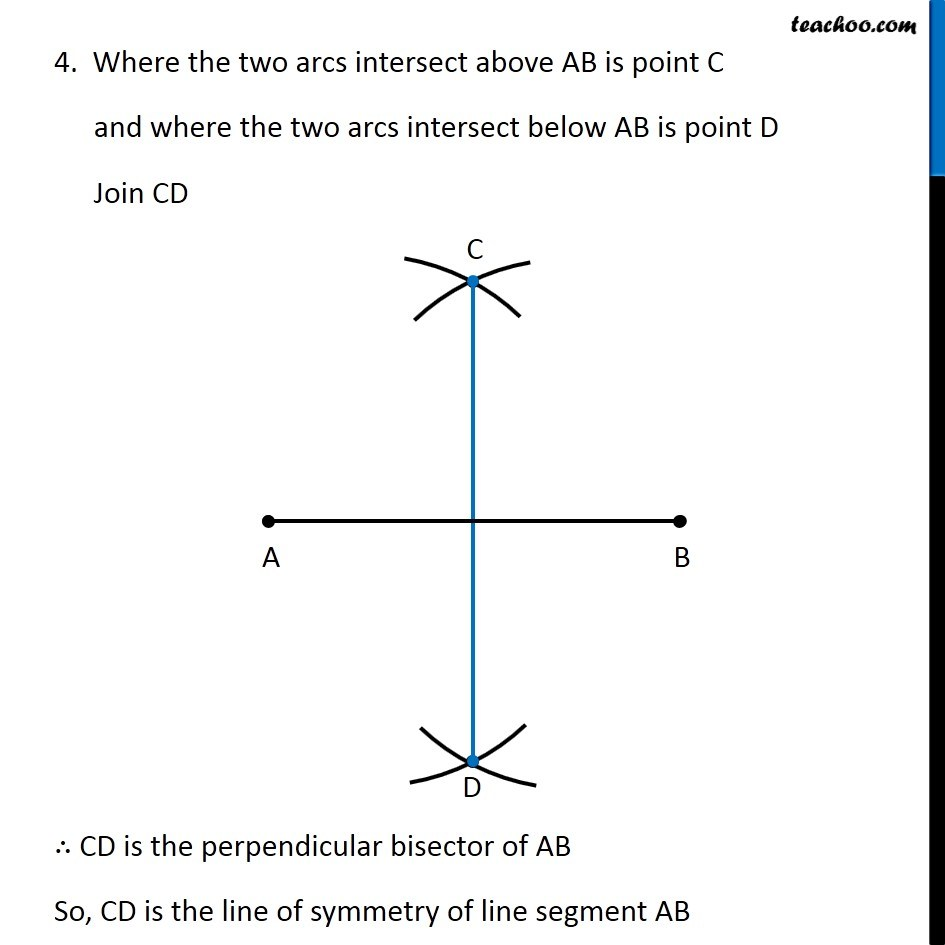Learn in your speed, with individual attention - Teachoo Maths 1-on-1 Class

### Transcript

Question 1 Draw (𝐴𝐵) ̅ of length 7.3 cm and find its axis of symmetry. Let’s first draw a line segment of length 7.3 cm We follow these steps 1. Draw a line l mark point A on it 2. Since length is 7.3 cm, we measure 7.3 cm using ruler and compass 3. Now keeping compass opened the same length. We keep pointed end at point A, and draw an arc on line l So, the required line segment is (𝐴𝐵) ̅ = 7.3 cm Now, we need to find its axis of symmetry That is, we need to make its perpendicular bisector Let’s follow these steps to make perpendicular bisector of AB 1. Given a line segment AB. 2. Now with A as center, and radius more than half AB, draw an arc on top and bottom of AB 3. Now with B as center, and same radius, draw an arc on top and bottom of AB 4. Where the two arcs intersect above AB is point C and where the two arcs intersect below AB is point D Join CD ∴ CD is the perpendicular bisector of AB So, CD is the line of symmetry of line segment AB